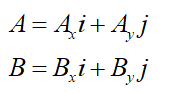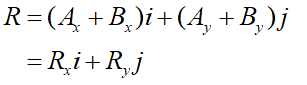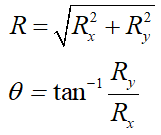# What is the difference between the graphical and component method of vector addition

Question
16 views

What is the difference between the graphical and component method of vector addition

check_circle

Step 1

A vector is a quantity that has both magnitude and direction.

Step 2

In this method, the vectors given are drawn to proper scale with proper direction. The second vector is drawn such that its tail falls at the tip of first one.

The resultant is drawn starting from the tip of second vector to the tail of 1st one. The magnitude and direction of this vector is measure with the help of scale and angle with protractor.

Step 3

In this case the two vectors are written asThe resultant is then given as R = A + B as,Magnitude of this vector is found as...

### Want to see the full answer?

See Solution

#### Want to see this answer and more?

Solutions are written by subject experts who are available 24/7. Questions are typically answered within 1 hour.*

See Solution
*Response times may vary by subject and question.
Tagged in

### Physics